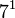# Groups of order 252

## Contents

See pages on algebraic structures of order 252| See pages on groups of a particular order

## Statistics at a glance

The number 252 has prime factors 2, 3, and 7. The prime factorization is:$\! 252 = 2^2 \cdot 3^2 \cdot 7^1 = 4 \cdot 9 \cdot 7$

All groups of this order are finite solvable groups. In particular, there is no simple non-abelian group of this order.

Quantity Value Explanation
Total number of groups up to isomorphism 46
Number of abelian groups up to isomorphism 4 (number of abelian groups of order$2^2$)$\times$ (number of abelian groups of order$3^2$)$\times$ (number of abelian groups of order$7^1$) = (number of unordered integer partitions of 2)$\times$ (number of unordered integer partitions of 2)$\times$ (number of unordered integer partitions of 1) =$2 \times 2 \times 1 = 4$. See classification of finite abelian groups and structure theorem for finitely generated abelian groups.
Number of nilpotent groups up to isomorphism 4 (number of groups of order 4)$\times$ (number of groups of order 9)$\times$ (number of groups of order 7) =$2 \times 2 \times 1 = 4$.
Number of supersolvable groups up to isomorphism 39
Number of solvable groups up to isomorphism 46 All groups of this order are solvable, i.e., the number 252 is a solvability-forcing number.
Number of simple groups up to isomorphism 0

## GAP implementation

The order 252 is part of GAP's SmallGroup library. Hence, any group of order 252 can be constructed using the SmallGroup function by specifying its group ID. Also, IdGroup is available, so the group ID of any group of this order can be queried.

Further, the collection of all groups of order 252 can be accessed as a list using GAP's AllSmallGroups function.

Here is GAP's summary information about how it stores groups of this order, accessed using GAP's SmallGroupsInformation function:

gap> SmallGroupsInformation(252);

There are 46 groups of order 252.
They are sorted by their Frattini factors.
1 has Frattini factor [ 42, 1 ].
2 has Frattini factor [ 42, 2 ].
3 has Frattini factor [ 42, 3 ].
4 has Frattini factor [ 42, 4 ].
5 has Frattini factor [ 42, 5 ].
6 has Frattini factor [ 42, 6 ].
7 has Frattini factor [ 84, 7 ].
8 has Frattini factor [ 84, 8 ].
9 has Frattini factor [ 84, 9 ].
10 has Frattini factor [ 84, 10 ].
11 has Frattini factor [ 84, 11 ].
12 has Frattini factor [ 84, 12 ].
13 has Frattini factor [ 84, 13 ].
14 has Frattini factor [ 84, 14 ].
15 has Frattini factor [ 84, 15 ].
16 has Frattini factor [ 126, 7 ].
17 has Frattini factor [ 126, 8 ].
18 has Frattini factor [ 126, 9 ].
19 has Frattini factor [ 126, 10 ].
20 has Frattini factor [ 126, 11 ].
21 has Frattini factor [ 126, 12 ].
22 has Frattini factor [ 126, 13 ].
23 has Frattini factor [ 126, 14 ].
24 has Frattini factor [ 126, 15 ].
25 has Frattini factor [ 126, 16 ].
26 - 46 have trivial Frattini subgroup.

For the selection functions the values of the following attributes
are precomputed and stored:
IsAbelian, IsNilpotentGroup, IsSupersolvableGroup, IsSolvableGroup,
LGLength, FrattinifactorSize and FrattinifactorId.

This size belongs to layer 2 of the SmallGroups library.
IdSmallGroup is available for this size.